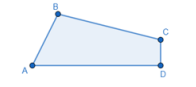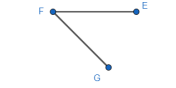# MA.912.GR.5.1Export Print
Construct a copy of a segment or an angle.

### Clarifications

Clarification 1: Instruction includes using compass and straightedge, string, reflective devices, paper folding or dynamic geometric software.
General Information
Subject Area: Mathematics (B.E.S.T.)
Strand: Geometric Reasoning
Status: State Board Approved

## Benchmark Instructional Guide

• Angle

### Vertical Alignment

Previous Benchmarks

Next Benchmarks

### Purpose and Instructional Strategies

In elementary grades, students drew lines and angles using a variety of tools, including rulers and protractors. In Geometry, students are introduced to constructions for the first time, specifically copying a segment or an angle. These two procedures are embedded in other basic constructions, and the concept of constructing and identifying copies of segments and angles is closely connected to visualizing and understanding congruence.
• Instruction includes the use of manipulatives, tools and geometric software. Allowing students to explore constructions with dynamic software reinforces why the constructions work.
• For example, students can use tracing/folding paper (e.g., patty paper) to trace the copy of an angle, or the copy of a segment, and verify that the angle and its copy are congruent, or that the segment and its copy are congruent. Additionally, using several folds, it is possible to verify the congruency of two angles or two segments drawn on the same piece of paper.
• Instruction includes the connection to logical reasoning and visual proofs when verifying that a construction works.
• Instruction includes discussing the role of the compass in a geometric construction, beyond drawing circles, and how a string can replace a compass. Most of the time in this course, compasses will be used to draw arcs. Discuss how no matter the point chosen on the arc, the distance to the given point is the same.
• For example, students can place the compass at P and draw an arc. Choosing two points on the arc, A and B, the distance to P is the same, AP = BP and AP ≅ BP are radii of the circle containing the drawn arc centered at P
• Instruction includes the student understanding that in a geometric construction, one does not use the markings on a ruler or on a protractor to copy a segment or angle. Students should realize that there are limitations on precision that are inherent in the markings on rulers or protractors.
• It is important to build the understanding that formal constructions are valid when the lengths of segments or measures of angles are not known, or have values that do not appear on a ruler or protractor, including irrational values.
• For expectations of this benchmark, constructions should be reasonably accurate and the emphasis is to make connections between the construction steps and the definitions, properties and theorems supporting them.
• While going over the steps of geometric constructions, ensure that students develop vocabulary to describe the steps precisely. (MTR.4.1
• Problem types include identifying the next step of a construction, a missing step in a construction or the order of the steps in a construction.

### Common Misconceptions or Errors

• Students may not understand that the size of the angle, “the opening,” is what is being measured when copying an angle.
• Students may not understand why they are not using the marking on rulers and protractors to copy segments and angles.

• Create a construction of quadrilateral JKLM so that it is congruent to quadrilateral ABCD.• Given angle EFG below, create a copy so that it creates parallelogram EFGH.### Instructional Items

Instructional Item 1
• Construct the necessary segments and angles to construct quadrilateral EFGH so that it is congruent to quadrilateral EFGH. Assume ∠DAB ≅ ∠EFG,  DA ≅ EF and AB ≅ FG.*The strategies, tasks and items included in the B1G-M are examples and should not be considered comprehensive.

## Related Courses

This benchmark is part of these courses.
1200400: Foundational Skills in Mathematics 9-12 (Specifically in versions: 2014 - 2015, 2015 - 2022, 2022 and beyond (current))
1206310: Geometry (Specifically in versions: 2014 - 2015, 2015 - 2022, 2022 and beyond (current))
1206320: Geometry Honors (Specifically in versions: 2014 - 2015, 2015 - 2022, 2022 and beyond (current))
1206315: Geometry for Credit Recovery (Specifically in versions: 2014 - 2015, 2015 - 2022, 2022 and beyond (current))
7912065: Access Geometry (Specifically in versions: 2015 - 2022, 2022 and beyond (current))

## Related Access Points

Alternate version of this benchmark for students with significant cognitive disabilities.
MA.912.GR.5.AP.1: Construct a copy of a segment.

## Related Resources

Vetted resources educators can use to teach the concepts and skills in this benchmark.

## Formative Assessments

Constructing a Congruent Angle:

Students are asked to construct an angle congruent to a given angle.

Type: Formative Assessment

Constructing a Congruent Segment:

Students are asked to construct a line segment congruent to a given line segment.

Type: Formative Assessment

## Original Student Tutorials

Angle UP: Player 1:

Explore the construction processes for constructing an angle bisector, copying an angle and constructing a line parallel to a given line through a point not on the line using a variety of tools in this interactive, retro video game-themed tutorial.

NOTE: This tutorial uses both the angle bisector construction and the construction to copy an angle as an extension opportunity to also construct a line parallel to a given line through a point not on the line. Students also learn to identify corresponding angles created when a transversal crosses parallel lines, and discover using Geogebra that these angles are congruent.

Type: Original Student Tutorial

Meet Me Half Way:

Plan a paddle board expedition by learning how to do basic geometric constructions including copying a segment, constructing a segment bisector, constructing a segment's perpendicular bisector and constructing perpendicular segments using a variety of tools in this interactive tutorial.

Type: Original Student Tutorial

## MFAS Formative Assessments

Constructing a Congruent Angle:

Students are asked to construct an angle congruent to a given angle.

Constructing a Congruent Segment:

Students are asked to construct a line segment congruent to a given line segment.

## Original Student Tutorials Mathematics - Grades 9-12

Angle UP: Player 1:

Explore the construction processes for constructing an angle bisector, copying an angle and constructing a line parallel to a given line through a point not on the line using a variety of tools in this interactive, retro video game-themed tutorial.

NOTE: This tutorial uses both the angle bisector construction and the construction to copy an angle as an extension opportunity to also construct a line parallel to a given line through a point not on the line. Students also learn to identify corresponding angles created when a transversal crosses parallel lines, and discover using Geogebra that these angles are congruent.

Meet Me Half Way:

Plan a paddle board expedition by learning how to do basic geometric constructions including copying a segment, constructing a segment bisector, constructing a segment's perpendicular bisector and constructing perpendicular segments using a variety of tools in this interactive tutorial.

## Student Resources

Vetted resources students can use to learn the concepts and skills in this benchmark.

## Original Student Tutorials

Angle UP: Player 1:

Explore the construction processes for constructing an angle bisector, copying an angle and constructing a line parallel to a given line through a point not on the line using a variety of tools in this interactive, retro video game-themed tutorial.

NOTE: This tutorial uses both the angle bisector construction and the construction to copy an angle as an extension opportunity to also construct a line parallel to a given line through a point not on the line. Students also learn to identify corresponding angles created when a transversal crosses parallel lines, and discover using Geogebra that these angles are congruent.

Type: Original Student Tutorial

Meet Me Half Way:

Plan a paddle board expedition by learning how to do basic geometric constructions including copying a segment, constructing a segment bisector, constructing a segment's perpendicular bisector and constructing perpendicular segments using a variety of tools in this interactive tutorial.

Type: Original Student Tutorial

## Parent Resources

Vetted resources caregivers can use to help students learn the concepts and skills in this benchmark.